# Quadrilaterals Solution of TS & AP Board Class 9 Mathematics

###### Exercise 8.1

Question 1.

State whether the statements are True or False.

(i) Every parallelogram is a trapezium ( )

(ii) All parallelograms are quadrilaterals ( )

(iii) All trapeziums are parallelograms ( )

(iv) A square is a rhombus ( )

(v) Every rhombus is a square ( )

(vi) All parallelograms are rectangles ( )

(i) [True]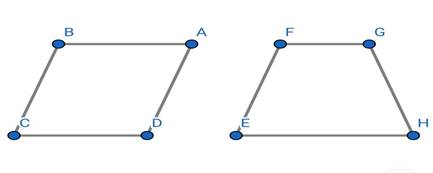⇒ The trapezium has a property

One pair of opposite sides are parallel

This is conveyed by parallelogram

as they have 2 pair of parallel sides

∴ Every parallelogram is a trapezium

(ii) [True]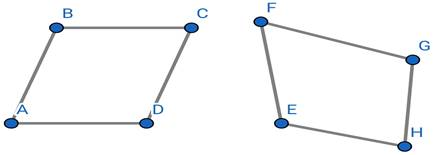⇒ The quadrilateral is known as

Polygon having four sides

This is conveyed by parallelogram

as they are also four sided polygon.

∴ Every parallelogram is a Quadrilateral

(iii) [False]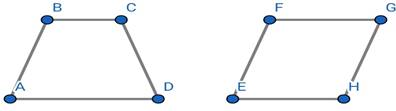⇒ The parallelogram has a property

Both pair of opposite sides are parallel and equal

Which is not conveyed by trapezium

as they have only 1 pair of parallel sides

∴ Every trapezium is not parallelogram

(iv) [True]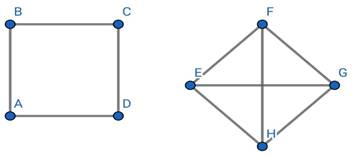⇒ The Rhombus has a property

Diagonal of rhombus bisect each other at 90°

This is conveyed by square

as their diagonal also bisect each other at 90°

∴ A square is a rhombus

(v) [False]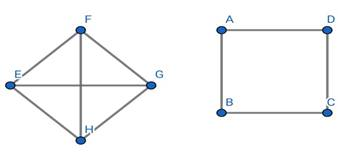⇒ The square has a property

All angles of square are equal and 90°

And diagonals are equal and perpendicular bisector to each

other

Which is not conveyed by Rhombus

as their diagonal are perpendicular bisector but not equal

And their angles are also not equal to 90°

∴ Every rhombus is not a square.

(vi) [False]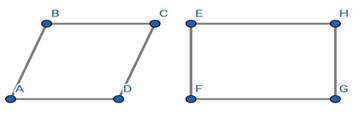⇒ The rectangle has a property

Every angle of rectangle is 90°

Which is not conveyed by parallelogram

as it have only opposite side angles are equal not 90°

∴ Every parallelogram are not rectangle.

Question 2.

Complete the following table by writing (YES) if the property holds for the particular Quadrilateral and (NO) if property does not holds.Question 3.

ABCD is trapezium in which AB || CD. If AD = BC, show that ∠A = ∠B and ∠C = ∠D.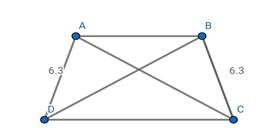Given:- ABCD is a isosceles trapezium

Trapezium with one pair of sides is parallel

And other pair of sides is equal[AD=BC].

Formula used:- SSS congruency property

If all 3 sides of triangle are equal to all 3 sides of

other triangle

Then; Both triangles are congruent

Solution:- In trapezium ABCD

If AB||CD

∴ ABCD is a isosceles trapezium

⇒ If ABCD is a isosceles trapezium

Then;

Diagonals of ABCD must be equal

∴ AC=BD

In Δ ABD and Δ ABC

AC=BD [∵ ABCD is isosceles trapezium]

AB=AB [Common line in both triangle]

∴ Both triangles are congruent by SSS property

∆ABD ≅ ∆ABC

⇒ If both triangles are congruent

Then there were also be equal.

∴ ∠ A=∠ B

As ABCD is trapezium and AB||CD

∠ A+∠ D=180° and ∠ C+∠ B=180°

∠ A =180° - ∠ D and ∠ B=180° - ∠ C

If ∠ A=∠ B;

180° - ∠ D=180° - ∠ C

180° +∠ C - 180° = ∠ D

∴ ∠ C=∠ D;

Conclusion:- If ABCD is trapezium in which AD = BC, then ∠A = ∠B and ∠C = ∠D.

Question 4.

The four angles of a quadrilateral are in the ratio 1: 2:3:4. Find the measure of each angle of the quadrilateral.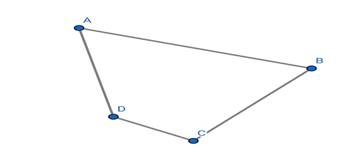Given . Angles of a quadrilateral are in the ratio 1: 2:3:4

Solution .

Then

Sum of all angles of quadrilateral is 360°

∴ ∠ A + ∠ B + ∠ C + ∠ D=360°

If angle A,B,C,D are in ratio 1:2:3:4

Then,

1x + 2x + 3x + 4x =360°

10x=360°

x=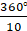x= 36°

all angles are 1x,2x,3x,4x

Conclusion.

∴ Angles are 36° , 72° , 108° , 144°

Question 5.

ABCD is a rectangle AC is diagonal. Find the angles of ∆ACD. Give reasons.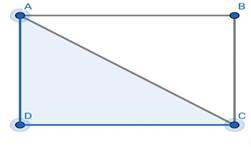Given:- ABCD is a rectangle

Formula used:- All angles of rectangle are 90°

In right angle triangle

Pythagoras theorem a2+b2=c2

Sin(θ)=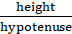Solution:-

In ΔACD

∠ D=90° [All angles of rectangle are 90° ]

∠ ACD=θ1

And; Sin(θ1) =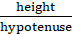=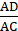θ1=arc sin()

∠ DAC=θ2

And; Sin(θ2) ==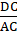θ1=arc sin(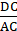)

Conclusion:-

The angles of Δ ACD are :-

∠ D=90°

∠ DAC=arc sin()

∠ ACD=arc sin(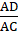)

###### Exercise 8.2

Question 1.

In the adjacent figure ABCD is a parallelogram ABEF is a rectangle show that ∆AFD ≅ ∆BEC.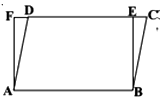Given :- ABCD is a parallelogram and ABEF is a rectangle

Formula used :-

SAS congruency rule

If two sides of triangle and angle made by the 2 sides are equal then both the triangles are congruent

Solution :-

In ∆AFD and ∆BEC

*AF=BE [opposite sides of rectangle are equal]

*AD=BC [opposite sides of parallelogram are equal]

As angle of rectangle is 90°

∠ DAB=90° - ∠ FAD -----1

Sum of corresponding angles of parallelogram is 180°

∠ DAB+∠ ABC =180°

∠ DAB+∠ ABE+∠ EBC=180°

90° - ∠ FAD + 90° +∠ EBC=180° ∵ putting value from 1

⇒ Hence;

By SAS property both triangles are congruent

Conclusion :-

∆AFD ≅ ∆BEC

Question 2.

Show that the diagonals of a rhombus divide it into four congruent triangles.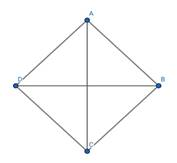Given :- ABCD is a rhombus

Formula used :-

*SSS congruency rule

If all sides of both triangles are equal then both triangles are congruent

*properties of rhombus

Solution :-

In Δ AOD and Δ COB

AO=OC [diagonal of Rhombus bisect each other]

OD=OB [diagonal of Rhombus bisect each other]

AD=BC [all sides of rhombus are equal]

∴ Δ AOD ≅ Δ COB

In Δ AOB and Δ COD

AO=OC [diagonal of Rhombus bisect each other]

OD=OB [diagonal of Rhombus bisect each other]

CD=BA [all sides of rhombus are equal]

∴ Δ AOB ≅ Δ COD

In Δ AOB and Δ AOD

AO=AO [Common in both triangles]

OD=OB [diagonal of Rhombus bisect each other]

AD=AB [all sides of rhombus are equal]

∴ Δ AOB ≅ Δ AOD

∴ All four triangles divide by diagonals of triangle are congruent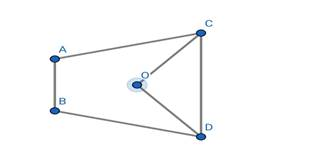Given :- ABCD is a quadrilateral

∠ OCB= ∠ OCD=∠C/2 [OC is bisector of ∠ C]

∠ ODA= ∠ ODC=∠D/2 [OD is bisector of ∠ D]

Formula Used:- ∠ A+ ∠ B+∠ C+ ∠ D=360°

Solution :-

In Δ COD

∠ OCD+ ∠ COD +∠ ODC=180°

∠D/2 + ∠C/2 +∠ COD = 180°

(∠ D+∠ C)/2 + ∠ COD = 180°

If;

∠ A+ ∠ B+∠ C+ ∠ D=360°

∠ C+ ∠ D=360° -(∠ A+ ∠ B)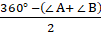+ ∠ COD=180°

180° -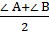+ ∠ COD=180°

∠ COD=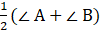###### Exercise 8.3

Question 1.

The opposite angles of a parallelogram are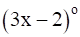and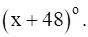Find the measure of each angle of the parallelogram.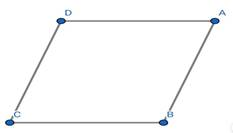Given:- Opposite angles of parallelogramandFormula used:- opposite angles of a parallelogram are equal

Corresponding angles sum is 180°

Solution:-

Let ∠ A and ∠ C

∠ A = ∠ C [opposite angles of a parallelogram are equal]

3x-2=x+48

3x – x=48+2

2x=50

x=25

Then;

∠ A=∠ C=x+48=25+ 48 = 73°

∠ A+∠ B=180°

∠ B=180° - 73°

∠ B= 107°

∠ B = ∠ D [opposite angles of a parallelogram are equal]

Conclusion:-

Angles of parallelogram = 73°, 107°, 73°, 107°

Question 2.

Find the measure of all the angles of a parallelogram, if one angle is 24° less than the twice of the smallest angle.Given:- one angle is 24° less than the twice of the smallest angle

Formula used:- opposite angles of a parallelogram are equal

Corresponding angles sum is 180°

Solution:-

Let ∠ A and ∠ C be smallest because

∠ A = ∠ C [opposite angles of a parallelogram are equal]

2∠ A+ 24° =∠ B

∠ A+∠ B=180° [Sum of corresponding angles is 180° ]

∠ B=180°-∠ A

Then,

2∠ A+ 24° =180° -∠ A

3∠ A =180° - 24°

3∠ A=156°

∠ A=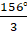=52°

∠ B=180° -∠ A

∠ B= 180° -52°

∠ B=128°

∠ B = ∠ D [opposite angles of a parallelogram are equal]

Conclusion:-

∴ Angles of parallelogram = 52°, 128°, 52°, 128°

Question 3.

In the adjacent figure ABCD is a parallelogram and E is the midpoint of the side BC. If DE and AB are produced to meet at F, show that AF = 2AB.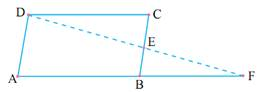Given :- ABCD is a parallelogram

And CE=EB

Formula used:- ASA congruency property

If 2 angles and one side between them in two

triangles are equal then both triangle are congruent

Solutions :-

In Δ DCE and Δ FBE

∠ DEC=∠ BEF [Vertically opposite angles]

As DC || BF

∠ ECD=∠ EBF [Alternate angles]

And;

CE=EB [Given]

∴ Δ DCE ≅ Δ FBE

As Δ DCE ≅ Δ FBE

Then

DC=BF

And DC=AB [opposite sides of parallelogram are equal]

Hence ;

AB=BF

AF=AB+BF

AF=2AB.

Hence Proved;

Question 4.

In the adjacent figure ABCD is a parallelogram P, Q are the midpoints of sides AB and DC respectively. Show that PBCQ is also a parallelogram.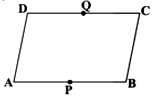Given:-ABCD is a parallelogram

P,Q are the midpoints of sides AB and DC respectively.

Solution:-

In parallelogram ABCD

If P,Q are the midpoints of sides AB and DC respectively

∴ DC=2QC=2QD

∴ AB=2AP=2PB

If DC||AB

Then;

QD||AP and QC||PB

Line PQ divides the parallelogram in 2 equal parts

Because P and Q are midpoints

If;

PQ||CB and QC||PB

Then, PBCQ is also a parallelogram

Question 5.

ABC is an isosceles triangle in which AB = AC. AD bisects exterior angle QAC and CD || BA as shown in the figure. Show that

(i) ∠DAC = ∠BCA

(ii) ABCD is a parallelogram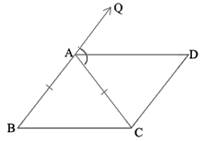Given:- AB=AC and ABC is isosceles triangle

CD||BA

Formula used:- sum of angles of triangle is 180°

Solution:-

As Δ ABC is a isosceles triangle

∠ ABC=∠ ACB

∠ ABC+∠ ACB+∠ CAB=180°

2∠ ACB+∠ CAB=180°

*As BAQ is a straight line

∠ CAB=180° - 2∠ DAC

Putting value of ∠ CAB in above equation 1

2∠ ACB+180° - 2∠ DAC=180°

2∠ ACB =2∠ DAC

∠ ACB =∠ DAC

If;

There is ∠ ACB =∠ DAC and AC is the transverse

∴ these are equal by alternate angles

In ABCD

If;

If both pair of sides of quadrilateral are parallel

Conclusion:-

ABCD is a parallelogram

And ∠DAC = ∠BCA

Question 6.

ABCD is a parallelogram AP and CQ are perpendiculars drawn from vertices A and C on diagonal BD (see figure) show that

(i) Δ APB ≅ Δ CQD

(ii) AP = CQ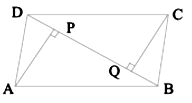Given:- ABCD is parallelogram

Formula used :- AAS property

If 2 angles and any one side is are equal in both triangle

Then both triangles are congruent

Solution:-

In Δ APB and Δ CQD

∠ CQD=∠ APB=90°

If ABCD is a parallelogram

∠ CDB=∠ DBA [alternate angles as DC||AB]

AB=DC [opposite sides of parallelogram are equal]

By AAS property

∴ Δ APB ≅ Δ CQD

If Δ APB ≅ Δ CQD

Then

AP=CQ

Conclusion:-

In parallelogram ABCD; Δ APB ≅ Δ CQD

Question 7.

In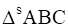and DEF, AB || DE, AB=DE; BC = EF and BC || EF. Vertices A, B and C are joined to vertices D, E and F respectively (see figure). Show that

(i) ABED is a parallelogram

(ii) BCFE is a parallelogram

(iii) AC = DF

(iv) ∆ABC ≅ ∆DEF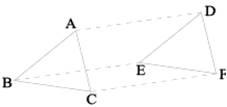Given:- AB||DE , AB=DE; BC||EF, BC=EF

Formula used:- SSS congruency rule

If all 3 sides of both triangle are equal then

Both triangles are congruent.

Solution:-

(1) In ABED

AB||DE

AB=DE

∴ ABED is a parallelogram

∵ If one pair of side in quadrilateral is equal and parallel

(2) In BCFE

BC||EF

BC=EF

∴ BCEF is a parallelogram

∵ If one pair of side in quadrilateral is equal and parallel

(3) As ABED is a parallelogram

As BCFE is a parallelogram

BE||CF , BE=CF ;

∴ By concluding both above statements

∴ ACFD is a parallelogram

∵ If one pair of side in quadrilateral is equal and parallel

If ACDF is a parallelogram ;

Then

AC=DF [opposite sides of parallelogram are equal ]

(4) In Δ ABC and Δ DEF

AB=DE [Given]

BC=EF [Given]

AC=DF [Proved above]

Δ ABC ≅ Δ DEF [SSS congruency rule]

Question 8.

ABCD is a parallelogram. AC and BD are the diagonals intersect at O. P and Q are the points of tri section of the diagonal BD. Prove that CQ || AP and also AC bisects PQ (see figure).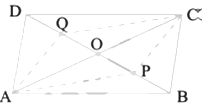Given:- ABCD is parallelogram

BP=BD/3

DQ=BD/3

Formula used:- SAS congruency property

If 2 sides and angle between the two sides of both

the triangle are equal, then both triangle are congruent

Solution:-

⇒ In parallelogram ABCD

AO=OC and DO=OB [diagonal of parallelogram bisect each other]

As DO=OB

Where DO=DQ+OQ

OB=OP+PB

∴ DQ+OQ=OP+PB

⇒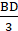+OQ=OP+⇒ OQ=OP+-⇒ OQ=OP

PQ = OP+OQ = 2(OQ) = 2(OP)

∴ AC diagonal bisect PQ

⇒ In Δ QOC and Δ POA

OQ=PO [Proven above]

AO=OC [Diagonal of parallelogram bisect each other]

∠ QOC=∠ AOP [vertically opposite angles]

Hence Δ QOC ≅ Δ POA

∴ ∠ CQO=∠ OPA

If QC and PA are 2 lines

And QP is the transverse

And ∠ CQO=∠ OPA by Alternate angles

∴ QC||PA

Conclusion:-

CQ||PA and CA is bisector of PQ.

Question 9.

ABCD is a square. E, F, G and H are the mid points of AB, BC, CD and DA respectively. Such that AE = BF = CG = DH. Prove that EFGH is a square.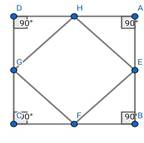Given:- *ABCD is a square

*E, F, G and H are the mid points of AB, BC, CD and DA

respectively

*AE = BF = CG = DH

Formula used:- *Isosceles Δ property

⇒ If 2 sides of triangle are equal then

Corresponding angle will also be equal

*Properties of quadrilateral to be square

⇒ All sides are equal

⇒ All angles are 90°

Solution:-

In Δ AHE, Δ EBF, Δ FCG, Δ DHG

⇒ AE = BF = CG = DH [Given]

If;

⇒ AE=EB [E is midpoint of AB]

⇒ BF=FC [F is midpoint of BC]

⇒ CG=GD [G is midpoint of CD]

⇒ DH=HA [H is midpoint of DA]

⇒ AE = BF = CG = DH

On replacing every part we get;

⇒ EB=FC=GD=HA;

⇒ ∠ A+∠ B+∠ C+∠ D=90° [All angles of square are 90°]

Hence;

All triangles Δ AHE, Δ EBF, Δ FCG, Δ DHG

are congruent by SAS property

∴ Δ AHE≅ Δ EBF≅ Δ FCG ≅ Δ DHG

⇒ HE=EF=FG=GH [All triangles are congruent]

In Δ AHE, Δ EBF, Δ FCG, Δ DHG

∵ all sides of square are equal and after the midpoint of each sides

Every half side of square are equal to half of other sides.

HA=AE , EB=FB ,FC=GC ,HD=DG

∴ All Δ AHE, Δ EBF, Δ FCG, Δ DHG are isosceles

⇒ as central angle of all triangle is 90°

It makes all Δ AHE, Δ EBF, Δ FCG, Δ DHG are right angle isosceles Δ

∴ all corresponding angles of equal side will be 45°

∠ AHE=∠ BEF=∠ CFG=∠ DHG=∠ AEH=∠ BFE=∠ CGF=∠ DGH=45°

⇒ as AB is straight line

Then; ∠ AEH+∠ HEF+∠ BEF=180°

45°+∠ HEF+45° =180°

∠ HEF=180° -90° =90°

Similarly ;

∠ EFG=90°

∠ FGH=90°

∠ GHE=90°

Conclusion:-

All angles are 90° and all sides are equal of quadrilateral

###### Exercise 8.4

Question 1.

ABC is a triangle. D is a point on AB such that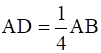and E is a point on AC such that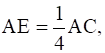If DE = 2 cm find BC.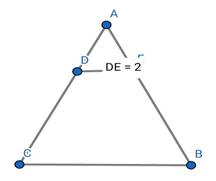Given:- DE=2cmFormula used:- Line joining midpoints of 2 sides of triangle will be

half of its 3rd side.

Solution:-

Assume mid points of line AB and AC is M and N

The formation of new triangle will be there Δ AMN

As;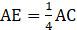⇒ AC=4AE

and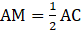∴ AM=2AE

Hence;

E is the midpoint of AM

Similarly

D is the midpoint of AN

→ In Δ AMN

If E,D are midpoints

ED=MN

MN=2ED

MN=2×2cm=4cm

→ In Δ ABC

If M,N are midpoints

AB=MN

AB=2MN

AB=2×4cm=8cm

Conclusion:-

BC=8cm

Question 2.

ABCD is quadrilateral E, F, G and H are the midpoints of AB, BC, CD and DA respectively. Prove that EFGH is a parallelogram.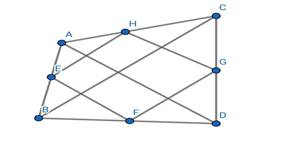Given:- ABCD is quadrilateral E, F, G and H are the midpoints of

AB, BC, CD and DA respectively

Formula used:- Line joining midpoints of 2 sides of triangle

Is parallel to 3rd side

Solution:-

EH is the line joined by midpoints of triangle ABD,

∴EH is parallel to BD

GF is line joined by midpoints of side BC&BD of triangle BCD

∴GF is parallel to BD

⇒ If HE is parallel to BD and BD is parallel to GF

∴ It gives HE is parallel to GF

⇒ AC is another diagonal of quadrilateral

GH is the line joined by midpoints of triangle ADC,

∴GH is parallel to AC

FE is line joined by midpoints of side BC&AB of triangle ABC

∴FE is parallel to AC

⇒ If GH is parallel to AC and AC is parallel to FE

∴ It gives GH is parallel to FE

As HE||GF and GH||FE

∴ EFGH is a parallelogram

Conclusion:-

EFGH is a parallelogram

Question 3.

Show that the figure formed by joining the midpoints of sides of a rhombus successively is a rectangle.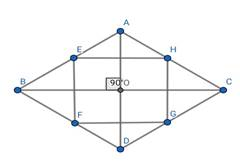Given:- ABCD is a rhombus

E,F,G,H are the mid points of AB,BC,CD,DA

Formula used:- Line joining midpoints of 2 sides of triangle

Is parallel and half of 3rd side

Solution:-

BD is diagonal of rhombus

EH is the line joined by midpoints of triangle ABD,

∴EH is parallel and half of BD

GF is line joined by midpoints of side BC&BD of triangle BCD

∴GF is parallel and half BD

⇒ If HE is parallel to BD and BD is parallel to GF

∴ It gives HE is parallel to GF

⇒ If HE is half of BD and GF is also half of BD

∴ It gives HE is equal to GF

⇒ AC is another diagonal of rhombus

GH is the line joined by midpoints of triangle ADC,

∴GH is parallel to AC

∴GH is half of AC

FE is line joined by midpoints of side BC&AB of triangle ABC

∴FE is parallel to AC

∴ FE is half of AC

⇒ If GH is parallel to AC and AC is parallel to FE

∴ It gives GH is parallel to FE

⇒ If GH is half of AC and FE is also half of AC

∴ It gives GH is equal to FE

As diagonals of rhombus intersect at 90°

And AC is parallel to FE and GH

All angles of EFGH is 90°

All angles are 90° and opposite sides are equal and parallel

Conclusion:-

EFGH is a rectangle

Question 4.

In a parallelogram ABCD, E and F are the midpoints of the sides AB and DC respectively. Show that the line segments AF and EC trisect the diagonal BD.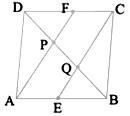Given:- ABCD is a parallelogram

E and F are the midpoints of the sides AB and DC respectively

Formula used:- Line drawn through midpoint of one side of triangle

Parallel to other side , bisect the 3rd side.

Solution:-

As ABCD is parallelogram

AB=CD and AB||CD;

⇒ AE=CF and AE||CF [E and F are the midpoints of AB and CD]

⇒ AE=CF and AE||CF

AECF is a parallelogram.

∵ Quadrilateral having one pair of side equal and parallel are parallelogram

If AECF is a parallelogram

∴ AF||CE

Hence ;

PF||CQ and AP||QE [As AF=AP+PF and CE=CQ+QE ]

In Δ DQC

DF=FC [F is midpoint]

PF||CQ

Then;

P is midpoint of DQ

DP=PQ

∵ Line drawn through midpoint of one side of triangle Parallel to

other side , bisect the 3rd side

In Δ APB

AE=EB [E is midpoint]

AP||QE

Then;

Q is midpoint of PB

PQ=QB

∵ Line drawn through midpoint of one side of triangle Parallel to

other side , bisect the 3rd side

If DP=PQ and PQ=QB

Then DP=PQ=QB

Conclusion:-

Line segments AF and EC trisect the diagonal BD.

Question 5.

Show that the line segments joining the midpoints of the opposite sides of a quadrilateral and bisect each other.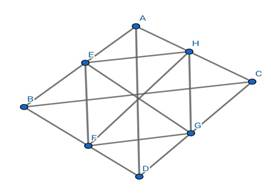Formula used:- Line joining midpoints of 2 sides of triangle

Is parallel and half of 3rd side

Solution:-

EH is the line joined by midpoints of triangle ABD,

∴EH is parallel and half of BD

GF is line joined by midpoints of side BC&BD of triangle BCD

∴GF is parallel and half BD

⇒ If HE is parallel to BD and BD is parallel to GF

∴ It gives HE is parallel to GF

⇒ If HE is half of BD and GF is also half of BD

∴ It gives HE is equal to GF

⇒ AC is another diagonal of quadrilateral

GH is the line joined by midpoints of triangle ADC,

∴GH is parallel to AC

∴GH is half of AC

FE is line joined by midpoints of side BC&AB of triangle ABC

∴FE is parallel to AC

∴ FE is half of AC

⇒ If GH is parallel to AC and AC is parallel to FE

∴ It gives GH is parallel to FE

⇒ If GH is half of AC and FE is also half of AC

∴ It gives GH is equal to FE

If both opposite sides are parallel and equal

If EFGH is parallelogram

Then their diagonal bisect each other

If diagonal of parallelogram is the line joining midpoint of opposite sides of quadrilateral

Then;

Line joining midpoints of opposite sides of quadrilateral bisect each other.

Conclusion:-

Lines joining midpoints of opposite sides of quadrilateral bisect each other

Question 6.

ABC is a triangle right angled at C. A line through the midpoint M of hypotenuse AB and Parallel to BC intersects AC at D. Show that

(i) D is the midpoint of AC

(ii) MD ⊥ AC

(iii) CM = MA =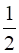AB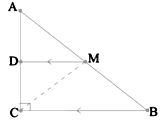Given:- ACB is right angle triangle

M is midpoint of AB

DM||CB

Formula used:- Line drawn through midpoint of one side of triangle

Parallel to other side , bisect the 3rd side

* SAS congruency property

If 2 sides and angle between the two sides of both

the triangle are equal, then both triangle are congruent

Solution:-

(1) In Δ ABC

M is midpoint of AB

And DM||CB

∴ D is midpoint of AC

∵ Line drawn through midpoint of one side of triangle

Parallel to other side , bisect the 3rd side

(2) In Δ ABC

DM||CB

∠ ACB =90°

(3) In Δ ADM and Δ DMC

⇒ DM=DM [Common in both triangles ]

⇒ AD=DC [D is the midpoint]

∠ MDC=90°

Hence;

In Δ ADM ≅ Δ DMC

∴ CM=MA

⇒ MA=AB [M is midpoint of AB]

∴ CM=MA=AB

## MATHS VIDEOS